## Sending data to Excel through the DDE interface. Tutorial.

Problem scenario:

The lab equipment has a RS232 port, which outputs a measurement data every 10 minutes. I would like to use parsed values in forms, formulas and my VBA logic in a Microsoft Excel spreadsheet.

Requirements:

It is assumed that:

You've configured communication parameters (baud rate, number of data bits, flow control etc) in the data logger and can receive any data without communication errors.

You can work in Microsoft Excel, specifically create and use forms.

Solution:

Note: This tutorial is based on the previous tutorial named "Serial port data and Excel. Real time charting". This example will use the parser settings and the data example from this tutorial. Therefore you may read it before continue.

The parser is ready we've tested it in the previous tutorial. If the parser had been correctly set up, then you should see all parser items names and their values (fig.1) in the DDE server window below.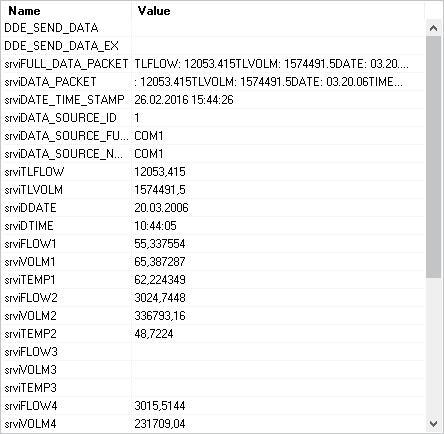Fig.1. Serial port data, Excel and DDE. DDE server window.

All parser items are now ready for usage from Microsoft Excel. The variable name is displayed in the "Name" column. You should know the DDE server name and the topic name to retrieve values via DDE. This information exists on the following page (fig.2 pos.1). Please, note that the server name may be different on your PC.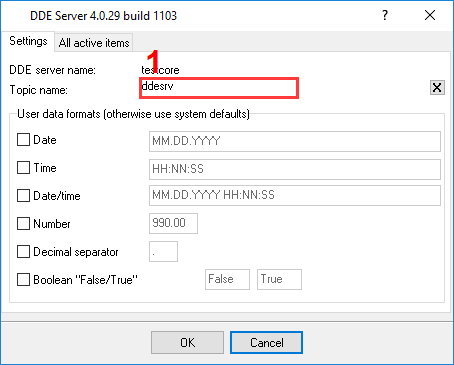Fig.2. Serial port data, Excel and DDE. DDE server parameters.

Click the "OK" button and close the DDE Server plug-in configuration window and the "OK" button in the options window.

Because we want to draw a chart in a real-time environment then we should prepare an Excel file before the next step. In this example we've created a diagram with 3 plots. Each plot is based on a data from columns A, B or C. We'll place FLOW1, VOLW1 and TEMP1 to a corresponding column and the chart will be automatically updated every time. We'll use last 30 values on the diagram only. In this example, we've placed 3 links to DDE items to cells D21-F21 like: "=testcore|ddesrv!srviFLOW1" (w/o quotes) (fig.3 and 4). As you can see, the server name, the topic name and the variable name are specified in this DDE link.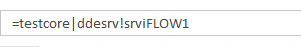Fig.3. Serial port data, Excel and DDE. DDE links.

After you've placed the DDE link to the spreadsheet Microsoft Excel will start the data logger and retrieve the data automatically. If the data logger is already started then Microsoft Excel will try to read the data from the existing instance. If the data logger didn't receive and didn't parse the data yet, then Microsoft Excel will display a text about the broken link, but will read a value after successful parsing.

We are now ready for creating a VBA code, which will process the DDE data. This code will copy DDE values to columns, move old values and add rows to the history list (fig.4, 5).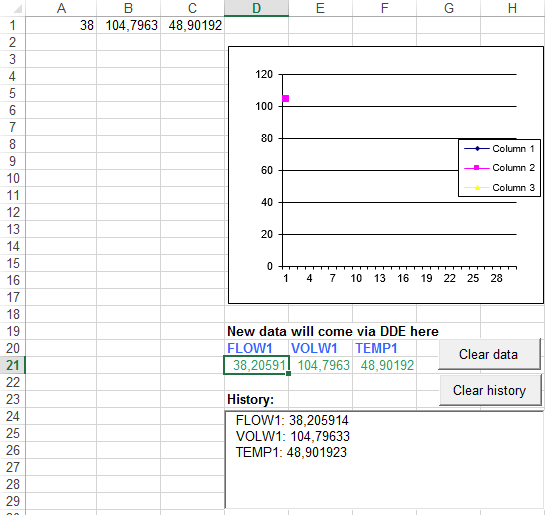Fig.4. Serial port data, Excel and DDE. Source spreadsheet.

The figure above contains following elements:

1. The DDE data will be placed here and it is a source for plots in this example;
2. Diagram;
3. History list.

VBA code from the Excel file

 #codewrap { overflow-x: scroll; overflow-y: scroll; overflow:auto; width: 550px; height: 350px; margin: 0px; padding: 5px; white-space: pre; font-size: 12px; border: 1px solid #666666; } ```Private Sub btnClear_Click() lbHistory.Clear 'MsgBox (CStr(Target.Row) + ":" + CStr(Target.Column)) End Sub Public Sub Process_Value(ByVal X_Coord As Long, ByVal ValueName As String, ByVal Value As Variant) Dim TmpValue As Variant Dim LastIndex As Long ' if we've read and non-numeric value, then replace it by ### in the grid If IsNumeric(Value) Then TmpValue = Value Else TmpValue = "####" End If ' search for an empty cell LastIndex = 0 For I = 1 To 30 If Cells(I, X_Coord).Value = "" Then LastIndex = I Exit For End If Next ' if we did not find an empty cell the move old value If LastIndex = 0 Then LastIndex = 30 For I = 2 To 30 Cells(I - 1, X_Coord).Value = Cells(I, X_Coord).Value Next End If ' place new value to the cell Cells(LastIndex, X_Coord).Value = TmpValue ' add new value to the history list lbHistory.AddItem (ValueName + ": " + CStr(TmpValue)) End Sub Private Sub btnClearData_Click() For I = 1 To 30 Cells(I, 1).Value = "" Cells(I, 2).Value = "" Cells(I, 3).Value = "" Next End Sub Private Sub btnClearHistory_Click() lbHistory.Clear End Sub Private Sub Worksheet_Calculate() On Error GoTo ErrHandler Application.EnableEvents = False Call Process_Value(1, "FLOW1", Cells(21, 4).Value) Call Process_Value(2, "VOLW1", Cells(21, 5).Value) Call Process_Value(3, "TEMP1", Cells(21, 6).Value) Application.EnableEvents = True ErrHandler: Application.EnableEvents = True End Sub ```

Okay, all settings have been completed and we are ready to capture data to an Excel file.

Connect your device and power it on if necessary. Check to see if you are able to receive a data block from the specified serial port. If the parser had been correctly set up, then you should see all parser items values (Fig.5) in the Excel spreadsheet.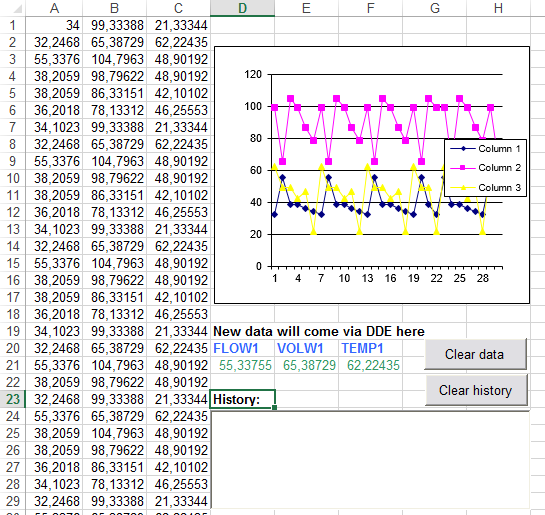Fig.5 Serial port data, Excel and DDE. Results.

The figure above contains following elements:

1. Last 30 values of the DDE data;
2. Last DDE values;
3. DDE data history.

The Excel file with all values and plots you can download here.

This method of retrieving data is complex and might prove to be difficult for the unskilled user, but allows you to process the data as you want. Later, you may make the following improvements in this example like:

1. Mark some values by color;
2. Add date/time stamp to the history list and auto-clear the list;
3. Use formulas and calculations.